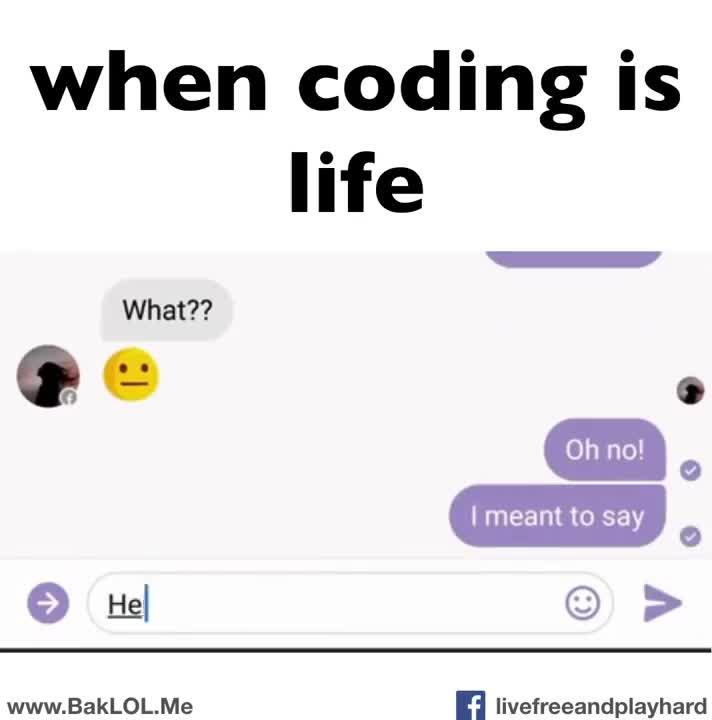# 作为程序员，这个七夕该怎么过？

## 程序员怎么过七夕？Crossin的编程教室 - Python新手村

1. 前谷歌工程师 Colin McMillen 用一段 perl 代码向他的女友 Kristen Stubbs 求婚：

``````#!/usr/bin/perl -w
use strict;

my\$f=           \$[;my
\$ch=0;sub       l{length}
sub r{join"",   reverse split
("",\$_[\$[])}sub ss{substr(\$_
,\$_,\$_)}sub be{\$_=\$_;p
(ss(\$_,\$f,1));\$f+=l()/2;\$f%=l
();\$f++if\$ch%2;\$ch++}my\$q=r
("\ntfgpfdfal,thg?bngbj".
"naxfcixz");\$_=\$q; \$q=~
tr/f[a-z]/ [l-za-k]
/;my@ever=1..&l
;my\$mine=\$q
;sub p{
print
@_;
}

be \$mine for @ever``````

``kristen, will you marry me?``

2. 在网页上发布以下文字：

``````99669999996669999996699666699666999966699666699
99699999999699999999699666699669966996699666699
99669999999999999996699666699699666699699666699
99666699999999999966666999966699666699699666699
99666666999999996666666699666699666699699666699
99666666669999666666666699666669966996699666699
99666666666996666666666699666666999966669999996``````

（来自网络）

3. 美国的一位工程师（严格来说这位不是程序员）Ben Kokes 亲手为女友打造了一枚“LED 发光戒指”。当他靠近女友时，女友手上的这枚的戒指就会发光。（袖子里藏了一个交变磁场发生器，而且得非常近）

4. 抖音表白神器：

5. 照片组字

6. 玫瑰曲线

``````import math
rad = 12
heart = '♥'
curve = []
for i in range(rad*2+1):
curve.append([])
for j in range(rad*2+1):
curve[i].append(' ')
for n in range(1,100):
print(n)
for k in range(360):
angle = k * math.pi / 180
x = int(rad * math.sin(n * angle) * math.sin(angle)) + rad
y = int(rad * math.sin(n * angle) * math.cos(angle)) + rad
curve[x][y] = heart
for i in range(rad*2+1):
for j in range(rad*2+1):
print(curve[i][j], end=' ')
curve[i][j] = ' '
print()``````

7. 由 0 和 1 组成的爱心图案（也有人说像屁股……）

``love.hackerzhou.me``

``hackerzhou.me/ex_love``When coding is Lifehttps://www.zhihu.com/video/1142446330503950336

（来源：bitbucket Spoon bitbucket.org/spooning/

## 扫描二维码下载知乎日报